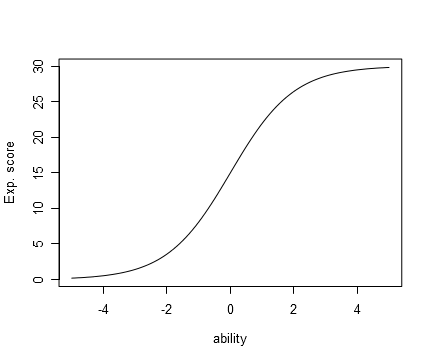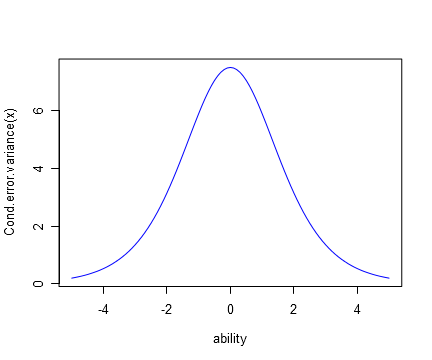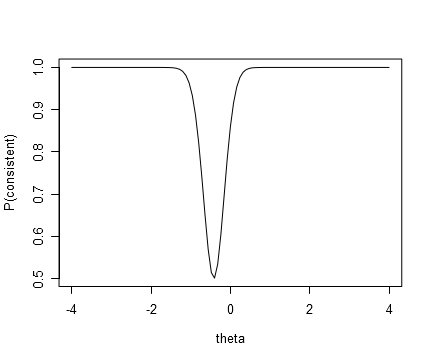There is a long-standing interest in combining item response theory (IRT) and classical test theory (CTT) rather than treat them as mere alternatives (Bechger et al. 2003). The theta functions in dexter are particularly helpful in this approach:

• expected_score(): The expected score given theta.
• information(): The Fisher information about theta in the test score.
• p_score(): The distribution of test scores given theta.

where theta, i.e., $$\theta$$, represents student ability. The aim of this blog is to illustrate the use of these functions but we commence with a (very) brief overview of CTT.

## Classical test theory

The test score is assumed to be a discrete random variable $$X_+$$ where the randomness is due to measurement error. The expected score of a test taker with ability $$\theta$$, $$\mathcal{E}[X_+|\theta]$$ is called the true score. It may be interpreted as the average score over (hypothetical) repeated testing of the same individual or as the average score of people with the same ability.

The true score is a function of ability and, in IRT parlance, would be called the test characteristic function (TCF). Obviously, the TCF cannot be observed but, if we assume a Rasch model, we can draw its curve using the function expected_score, the test score being the number of correct answers.

parms = data.frame(item_id=1:30, item_score=1, beta=rep(0,30))
Exp_sc = expected_score(parms)
plot(Exp_sc, from=-5,to=5, xlab="ability", ylab="Exp. score")The observed scores vary around their average due to measurement error, formally defined as deviations around the true score; $$x_+ - \mathcal{E}[X_+|\theta]$$. As we can always write $$x_+ = \mathcal{E}[X_+|\theta] + \left(x_+ - \mathcal{E}[X_+|\theta]\right)$$, we arrive at the famous equation: $\text{observed score} = \text{true score} + \text{measurement error}$ which is merely a tautology, equivalent to a random-effects ANOVA model.

The amount of measurement error at a given level of ability is captured by the conditional error variance (CEV): $\begin{equation} Var(X_+|\theta)=\mathcal{E}[\left(X_+ - \mathcal{E}[X_+|\theta] \right)^2|\theta] \end{equation}$ Like the true score, the CEV is a function of ability. Here is a plot.

Cond.error.variance = information(parms)
curve(Cond.error.variance, from=-5,to=5, col="blue", xlab="ability")Note that the CEV coincides with test information which is counter-intuitive as “error” sounds negative and “information” positive. Moreover, it is not true in general, but only in exponential family IRT models. We will clarify this in a future blog where we explain the difference between estimating a true score or an ability.

What happens in a population of test-takers? Using the law of total expectation, we find that, in a population of test-takers, the average of the true scores $$\mathcal{E}[\mathcal{E}[X_+|\theta]] = \mathcal{E}[X_+]$$ is just the average of the scores, as measurement errors average to zero. The variance of the test score is the sum of true score variance and error variance. Specifically, from the law of total variance it follows that: $Var(X_+)=Var(\mathcal{E}[X_+|\theta])+\mathcal{E}[Var(X_+|\theta)]$ where $$Var(\mathcal{E}[X_+|\theta])$$ is the true-score variance and \begin{align*} \mathcal{E}[Var(X_+|\theta)] & \equiv \mathcal{E}(\mathcal{E}[(X_+-\mathcal{E}[X_+|\theta])^{2}|\theta]) \\ &=\mathcal{E}[(X_+-\mathcal{E}[X_+|\theta])^{2}] \end{align*} is indeed the variance of the measurement errors, sometimes called the average error variance. In the population of test takers, measurement errors and true scores are uncorrelated but not necessarily independent. For one thing, we expect measurement error to be smaller for test takers whose true scores lie on the upper or lower end of the score range.

Last, but not least, the proportion true-score variance in the population is the reliability. That is, $\operatorname{rel}(X_+) \equiv\frac{\operatorname{Var}(\mathcal{E}[X_+|\theta])}{\operatorname{Var}(X_+)} =1-\frac{\mathcal{E}[\operatorname{Var}(X_+|\theta)]}{\operatorname{Var}(\mathcal{E}[X_+|\theta])+\mathcal{E }[Var(X_+|\theta)]}$ defined if $$\operatorname{Var}(X_+)>0$$. Note that, since $$Var(X_+) \geq Var(\mathcal{E}[X_+|\theta])$$, $$0\leq \operatorname{rel}(X_+)\leq 1$$. Reliability is the correlation between two administrations of the same test to samples from the same population (see Bechger et al. (2003)) and is widely used as a measure of consistency and an index for test quality. In the parlance of ANOVA it would be called the intra-class correlation (see Snijders and Bosker (2011), 3.5).

## Numerical Integration

CTT is sometimes called a weak theory of measurement as it based on one assumption only, namely that the test-score is random. Ability was introduced as a random variable to represent the test taker and could be continuous or discrete, uni- or multivariate. Assuming an IRT model like the Rasch model makes the theory stronger. The downside is that conclusions depend on the model fitting the data. The advantage is that it allows us to do more. We mention one possibility.

Using IRT, reliability can be calculated using numerical or Monte-Carlo integration. Here is a small script based on Gauss-Hermite Quadrature, which is the default procedure when the ability distribution is normal; see e.g., Kolen, Zeng, and Hanson (1996).

library(statmod)
x = GH$nodes w = GH$weights

es = expected_score(parms)
info = information(parms)

expected.score = sum(es(x) * w)

true.variance = sum(es(x)^2 * w) - expected.score^2

error.variance = sum(info(x) * w)

Assuming a standard normal ability distribution we find: true.variance = 39.04. The (average) error variance is just the expected information, in this case equal to error.variance = 6.2. The reliability is found to be true.variance/(error.variance+true.variance)) = 0.86.

## The Consistency of Classifications

Now for something new. Consider an exam that students fail if their test score is below a pass-fail score $$c$$, and pass otherwise. Let us consider the probability that a person with the same ability obtains the same result over two exchangeable replications. Let $$I=(X_+\geq c)$$ be an indicator of passing. Then, the probability of a consistent outcome over two exchangeable replications of the exam is: \begin{align*} p(\text{Consistent}|\theta) &= p(I=1,I^*=1|\theta) + p(I=0,I^*=0|\theta) \\ &=\left(\sum_{s\geq c} p(X_+ =s|\theta)\right)^2 + \left(\sum_{s< c} p(X_+ =s|\theta)\right)^2 \end{align*} In our 2003 article, we have called this the test-characteristic decision function. Here is how to compute this function using dexter’s function p_score, which calculates the test score distribution given ability.

TCDF = function(parms, c)
{
function(theta)
{
p.score = p_score(parms)(theta)
s = 1:ncol(p.score) - 1

rowSums(p.score[,s<c])^2 + rowSums(p.score[,s>=c])^2
}
}

As a somewhat ludicrous illustration, consider the verbal aggression test as an examination: everybody with a score of 20 or more gets official recognition as a verbally aggressive person. We obtain:

plot(TCDF(parms,20), from=-4, to=4, xlab='theta',ylab='P(consistent)')Integrating the TCDC over the population that took the test (we have estimated that a normal distribution with mu=-0.8 and sigma=1 is a good choice), we obtain the probability of consistent classification when the test is applied to this population using c as a cutoff. For the verbal aggression test with a pass-fail score of 20 this is 0.86 – not a bad result. The following function performs the computation:

Cons_class = function(parms, c, mu=0, sigma=1)
{
sum(TCDF(parms, c)(GH$nodes) * GH$weights)
}